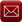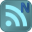You have downloaded this file 0 times in the last 24 hours, limit is 100.

## BEAMANAL (metric).xls This location is for Registered Users Only.Perhaps you need to login or register.

Description:
"BEAMANAL" is a spreadsheet program written in MS-Excel for the purpose of analysis of either single-span or continuous-span beams subjected to virtually any type of loading configuration.  Four (4) types of single-span beams and two (2) through (5) span, continuous-span beams, considered.  Specifically, beam end reactions as well as the maximum moments and deflections are calculated.  Plots of both the shear and moment diagrams are produced, as well as a tabulation of the shear, moment, slope, and deflection for the beam or each individual span.
Note: this is a metric units version of the original "BEAMANAL.xls" spreadsheet workbook.

This program is a workbook consisting of three (3) worksheets, described as follows:

Worksheet Name
Doc - This documentation sheet
Single-Span Beam - Single-span beam analysis for simple, propped, fixed, & cantilever beams
Continuous-Span Beam - Continuous-span beam analysis for 2 through 5 span beams

Program Assumptions and Limitations:
1.   The following reference was used in the development of this program (see below): "Modern Formulas for Statics and Dynamics, A Stress-and-Strain Approach" by Walter D. Pilkey and Pin Yu Chang, McGraw-Hill Book Company (1978), pages 11 to 21.
2.   This program uses the three (3) following assumptions as a basis for analysis:
a.  Beams must be of constant cross section (E and I are constant for entire span length).
b.  Deflections must not significantly alter the geometry of the problem.
c.  Stress must remain within the "elastic" region.
3.   On the beam or each individual span, this program will handle a full length uniform load and up to eight (8) partial uniform, triangular, or trapezoidal loads, up to fifteen (15) point loads, and up to four (4) applied moments.
4.   For single-span beams, this program always assumes a particular orientation for two (2) of the the four (4) different types.  Specifically, the fixed end of either a "propped" or "cantilever" beam is always assumed to be on the right end of the beam.
5.   This program will calculate the beam end vertical reactions and moment reactions (if applicable), the maximum positive moment and negative moment (if applicable), and the maximum negative deflection and positive deflection (if applicable).  The calculated values for the end reactions and maximum moments and deflections are determined from dividing the beam into fifty (50) equal segments with fifty-one (51) points, and including all of the point load and applied moment locations as well.  (Note: the actual point of maximum moment occurs where the shear = 0, or passes through zero, while the actual point of maximum deflection is where the slope = 0.)
6.   The user is given the ability to input two (2) specific locations from the left end of the beam to calculate the shear, moment, slope, and deflection.
7.   The user is also given the ability to select an AISC W, S, C, MC, or HSS (rectangular tube) shape to aide in obtaining the X-axis moment of inertia for input for the purely analysis worksheets.
8.   The plots of the shear and moment diagrams as well as the displayed tabulation of shear, moment, slope, and deflection are based on the beam (or each individual span) being divided up into fifty (50) equal segments with fifty-one (51) points.
9.   For continuous-span beam of from two (2) through five (5) spans, this program utilizes the "Three-Moment Equation Theory" and solves a system simultaneous equations to determine the support moments
10.  This program contains numerous “comment boxes” which contain a wide variety of information including explanations of input or output items, equations used, data tables, etc.  (Note:  presence of a “comment box” is denoted by a “red triangle” in the upper right-hand corner of a cell.  Merely move the mouse pointer to the desired cell to view the contents of that particular "comment box"). Calculation Reference
Beam calculation
Structural analysis
Submitted By:
Alex Tomanovich (ATomanovich)
Submitted On:
09 Oct 2009
File Size:
3,309.50 Kb
636
File Version:
1.1
File Author:
Alex Tomanovich
Rating:Total Votes:20
rairosh 7 months ago
ATomanovich 9 months ago

Robert,
Most likely you have not entered the loads in correctly. If you'd like, email me a copy of the example problem in question, and I will look it over.
Regards,
Alex
RobertRowley 12 months ago
It's perhaps me entering the loads wrong, but I cannot get the deflection to add up to excepted?
nawinphatt 10 years ago
goooooooooood
nawinphatt 10 years ago
goooooooooood
ATomanovich 10 years ago
In both of the calculation worksheets in the "BEAMANAL (metric).xls" workbook, I added the plots for both the slope (rotation) and deflection diagrams to compliment the shear and moment diagrams that were already included. This workbook is now version 1.1.

We have 352 guests and 5 members onlineEmail (preferred method of contact)US +1 617 5008224EU +44 113 8152220